## SOCNET@LISTS.UFL.EDU

#### View:

 Message:[First|Previous|Next|Last] By Topic:[First|Previous|Next|Last] By Author:[First|Previous|Next|Last] Font: Proportional Font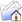LISTSERV Archives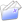SOCNET HomeSOCNET February 2011

Subject:From:Date:Wed, 23 Feb 2011 19:49:39 +0100

Content-Type:text/plain

Parts/Attachments: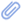text/plain (58 lines)
 ```***** To join INSNA, visit http://www.insna.org ***** Tad, do you imply that a solution is known for k = 1? Isn't this NP? --Moses 2011/2/23 <[log in to unmask]>: > *****  To join INSNA, visit http://www.insna.org  ***** > > Dear Socnet Members > > Let me address a  question to those of you who have been watching > developments  in GRAPH THEORY. > > Is the following statement true? > > 'No nontrivial complete set collection of invariants has yet been found > for directed graphs > > Definitions > > A (finite) DIRECTED GRAPH is a mathematical object of the form G=(X,R), > where X is a finite set (of points) and R is  a binary relation in X. > > Two digraphs G=(X,R) and G =(X ,R ) are ISOMORPHIC if there exists a 1-1 > mapping F of X onto X  such that xRy if and only if  F(x)R F(y), for any > x,y in X. > > A STRUCTURAL PARAMETER of a digraph is any mapping P which assigns a > numerical value to any digraph in such a way that  P(G)=P(G ) if G and G > are isomorphic. > > A COMPLETE SET OF INVARIANTS is any finite collection P1, ,Pk of > structural parameters such that, for any two digraphs G and G , the > equations  P1(G)=P1(G ), ,Pk(G)=Pk(G ) imply that G and G  are isomorphic. > > For any n, let  gn denote the number of equivalence classes of  the > isomorphism relation in  the set of digraphs with X={1, ,n}. The numbers > 1, ,gn can be used as  code symbols of equivalence classes. A 'trivial' > set of invariants is obtained by assigning to any G=(X,R) the number n of > points (n=|X| ) and an appropriate symbol from the range from 1 to gn. > Hence, by saying that a set of invariants is  NONTRIVIAL I mean that  the > values of P1, ,Pk for two n-point digraphs G and G   can be computed > without prior knowledge of the list of isomorphism classes. > > Tad Sozanski > www.cyf-kr.edu.pl/~ussozans/ > > _____________________________________________________________________ > SOCNET is a service of INSNA, the professional association for social > network researchers (http://www.insna.org). To unsubscribe, send > an email message to [log in to unmask] containing the line > UNSUBSCRIBE SOCNET in the body of the message. > _____________________________________________________________________ SOCNET is a service of INSNA, the professional association for social network researchers (http://www.insna.org). To unsubscribe, send an email message to [log in to unmask] containing the line UNSUBSCRIBE SOCNET in the body of the message.```

#### Search Archives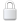Log In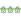Get Password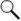Search Archives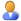Subscribe or Unsubscribe